## “ Gradient descent is a first-order iterative optimization algorithm for finding the minimum of a function. But, does it always work? What modifications can we make to adapt our gradient descent algorithm to different search spaces?”

Gradient descent is one of the most popular algorithms to perform optimization and by far the most common way to optimize artificial neural networks. It is often used as ‘Black-Box Optimizers’ but a good insight of various variations and mathematics behind them often comes handy. Gradient Descent can very well help in optimizing non-convex functions. But what if the contour (also known as our search space) generated by different functions is not so nice like Saddle points, valleys etc.

We can overcome most of the hurdles by building some concepts and bringing mathematical insights into its native implementation. There are three different implementations possible for our well known gradient descent algorithm:

• Batch Gradient Descent: This implementation is also known as Vanilla Gradient Descent. One of the basic steps in a gradient descent algorithm is the update step which updates the parameters of the Artificial Neural Networks.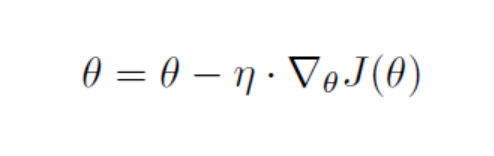θ = parameters

η = Learning rate

J = Cost Function

In Batch Gradient Descent, the parameters are updated only after the entire dataset has been traversed to calculate the cost of the gradient(J). So the main inference here is that it becomes relatively slow for Batch Gradient Descent algorithm to converge because it processes the entire dataset in a single update and also the ability of a model to learn online (when data is made available in sequential order) becomes difficult.

In SGD, updates in the parameters are performed after each training example. As we can notice, due to performing updates after each training example, the path to the optimum value is noisier and the minimum optimum is never reached practically. The function keeps oscillating around the minima due to high variance after each update.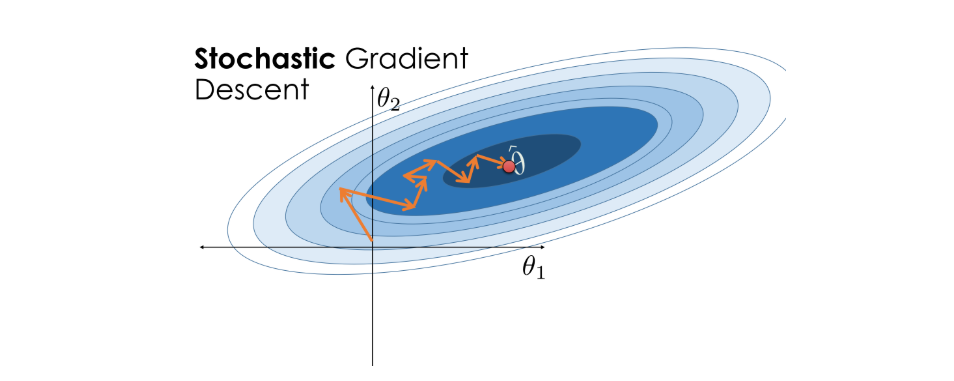Its advantage over Batch Gradient Descent is that it deals away with the redundant calculations on its way to minimum. As a result , it is much faster and can be used to learn through the data online.

Mini Batch Gradient maintains a balance between above two methods. In each iteration, now a fixed number of examples are chosen to make updates instead of making updates on a single example, i.e., a mini batch of examples are created and the learning rate is updated on each such iterations. The size of mini-batches is decided experimentally depending on the problems in hand and data is always shuffled before choosing the mini-batch of data.

Mathematically,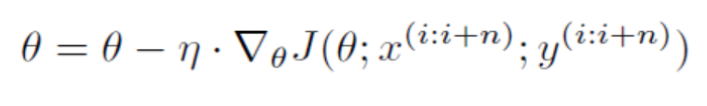θ = parameters

η = Learning rate

J = Cost Function

n = Number of training examples in a mini batch

Challenges involved in using these implementations of Gradient Descent:

• Choosing a proper learning rate is a challenge, if our learning rate is smaller this results in slower convergence of our loss function towards the minimum and can take larger amount of time, but if our learning rate is too large, this causes overshooting of the function away from the minimum, there are a lot of fluctuations and our function may diverge.

• The learning rate can be adjusted by trying Learning Rate Schedules during training where the learning rate is reduced when the objective function falls below a threshold value in an epoch, however these schedules have a problem of adapting to the characteristics of datasets as they have to be defined in advance. As we use the same learning rate to update all the parameters , the updating occurs by the same extent of all the parameters and we may not want this because if we have a sparse dataset with different relative frequencies of parameters we would want the rarely occurring parameters to be updated largely than the parameters which occur frequently.

• Another challenge for SGD Optimization is that it gets trapped in the so-called sub-optimal local minima in case of non-convex functions, also referred to as the saddle points, at this point the value of derivative is close to zero for a larger distance therefore the parameters are updated to a very less extent which implies very slow learning and becomes difficult to escape.

Considering these challenges in our conventional gradient descent algorithm, we propose some modifications to help the gradient descent algorithm perform well in different types of search spaces (Optimizers):

• Momentum:

The main problem SGD faces is while navigating around slopes of Ravine (Ravine is an area, where the surface curves much more steeply in one dimension than in another). It only makes hesitant progress along the bottom towards local optimum and makes a number of oscillations down its path. SGD with Momentum helps in overcoming large variations encountered on the way to optimum value. It acts as a means to dampen the oscillations and accelerate SGD in the relevant direction.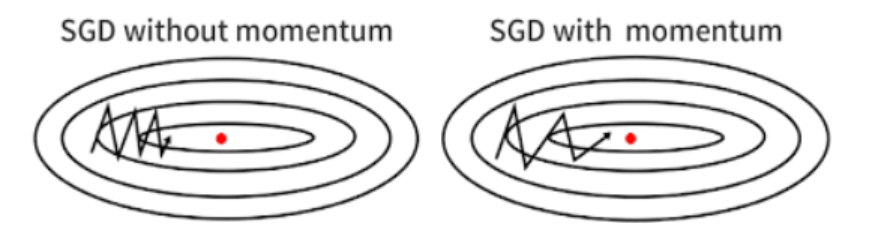https://distill.pub/2017/momentum/

The concept of Momentum is based on the idea of Exponentially Weighted Averages. It is a kind of moving average which would denoise the data and bring it closer to the original path the function would have followed in Batch Gradient Descent.

Mathematically the concept can be represented as: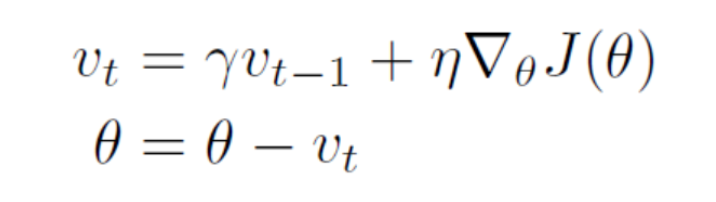Here, γ is the momentum term.

Intuitively, we can think of γ as : we are approximately averaging over the last 1 / (1- γ) points of the (sequence) path of the function.

In general γ is set to 0.9 or a similar value.

The momentum term increases for dimensions whose gradients point in the same directions and reduces updates for dimensions whose gradients change directions. As a result, we gain faster convergence and reduced oscillation.

This is similar to the Gradient Descent with momentum but we also introduce a new correction term in the update step.

Mathematically,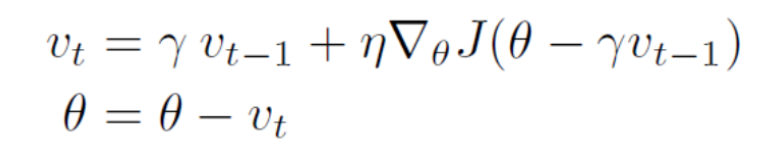Here in the Loss function the term “θ – γ.νₜ ₋₁” (Correction) helps in approximating the next updating process so that the oscillation is reduced.  With the help of Nesterov Accelerated Gradient, the function is able to make wise steps towards the minimum such that the number of oscillations is kept minimum.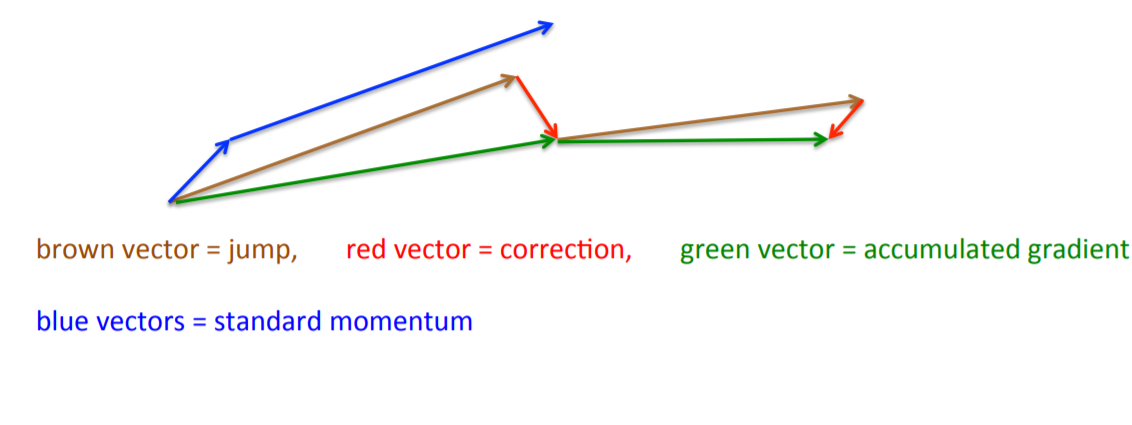NAG first makes a big jump in the direction of the previous accumulated gradient (brown vector), measures the gradient and then makes a correction (green vector), while Momentum first computes the current gradient (small blue vector) and then takes a big jump in the direction of the updated accumulated gradient (big blue vector). As a result, NAG helps in speeding up the convergence process and increases effectiveness of the algorithm by deciding whether to make a large update or small update on each iteration.

Tuning of the hyperparameters (like learning rate η) can be very difficult and may vary over different datasets. If we set η too small, then the parameter update will be very slow and it will take a very long time to converge or else if we set it too large, then the parameter will move all over the function and diverge away from the convergence point. To make things worse, the high-dimensional non-convex nature of an artificial neural networks optimization could lead to different sensitivity on each dimension. The learning rate could be too small in some dimension and could be too large in another dimension. Earlier an update was performed for all parameters θ at once as every parameter θᵢ used the same learning rate η. But AdaGrad uses uses a different learning rate for every parameter θᵢ at every time step t.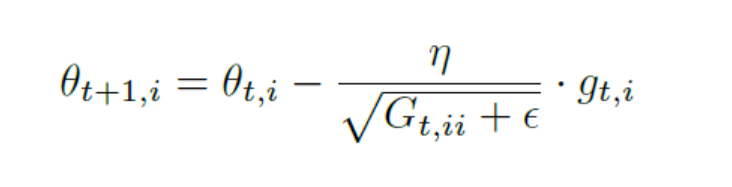Gₜ ∈ ℝᵈˣᵈ here is a diagonal matrix where each diagonal element i, i is the sum of the squares of the gradients w.r.t. θᵢ up to time step t.The matrix G is defined as Gₜ = ∑ gᵢ . gᵢ ᵀ where i varies from 1 to t. ϵ is a smoothing term that avoids division by zero (usually on the order of 1e⁻⁸). Actually, we can use the full matrix Gₜ in the parameter update, but computing the square root of the full matrix is impractical, especially in very high dimension. On the other hand, computing the square root and the inverse of only the diagonal diag(Gₜ) can easily be done. In this way AdaGrad does away with the manual setting of learning rate and we can set a default learning rate which is used throughout the learning process.

AdaDelta accumulates the gradients in the previous time steps using an exponentially decaying average of the squared gradients. This prevents the denominator from becoming infinitesimally small, and ensures that the parameters continue to be updated even after a large number of iterations. It also replaces the global learning rate η with an exponentially decaying average of the squares of the parameter updates over the previous iterations. This method has been shown to perform relatively well when used to train deep networks, and is much less sensitive to the choice of hyper-parameters.

Instead of inefficiently storing w previous squared gradients, the sum of gradients is recursively defined as a decaying average of all past squared gradients. The running average E[g²]ₜ at time step t then depends (as a fraction γ similarly to the Momentum term) only on the previous average and the current gradient:We now simply replace the matrix Gₜ by decaying gradients over the past squared gradients E[g²]ₜ :The value of gamma is generally set to around 0.9 same as we use in momentum. Also note that the update term above does not have the same units as that of the parameter, therefore we replace the learning rate n by the Root Mean Square(RMS) of the exponentially decaying averages of the squared parameter updates. Since, this RMS is not known for the current step , we replace it by the RMS of the previous step. In AdaDelta we do not even need to set the initial default value of learning rate since it has been replaced from the update rule.

• RMS Prop:

This algorithm also looks into the problems of the continuously diminishing gradient summation terms in the denominator of the update term, RMSprop as well divides the learning rate by an exponentially decaying average of squared gradients E[g²]. This step is similar to the first update step of AdaDelta. In general, default value of learning rate is chosen around 0.001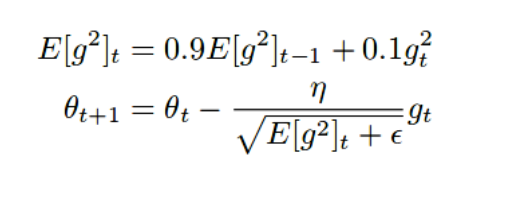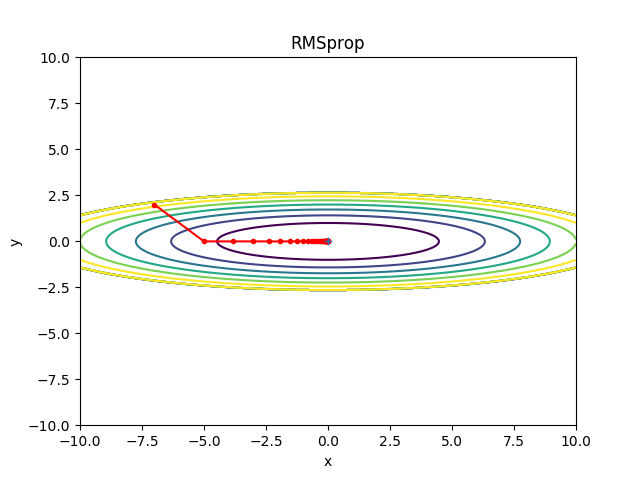This is also known as Adaptive Moment Estimation which calculates Adaptive Learning Rates for different parameters. It calculates the exponentially weighted average of the past gradients in addition to keeping track of the exponentially decaying averages of the past squared gradients.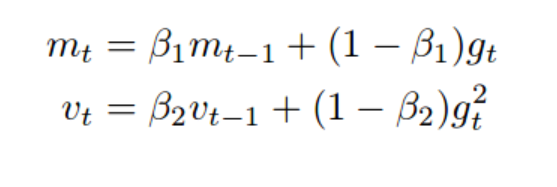where,

m ₜ = measures of the mean(first moment)

v ₜ = uncentered variance(second moment).

Since the vectors m and v here are initialized to zeros and the values of β1 and β2 are close to 1, their values are biased towards 0 during the initial steps when the decay rates are small. Therefore we introduce two bias correction terms and divide by them to counteract this problem, this term is large initially and becomes close to 1 as the steps become large. What basically β does is that it averages or smoothen the fluctuating curve by (1/1- β) terms. (For example if β = 0.9 , then it smoothens the curve by 1/(1-0.9) = 10 terms).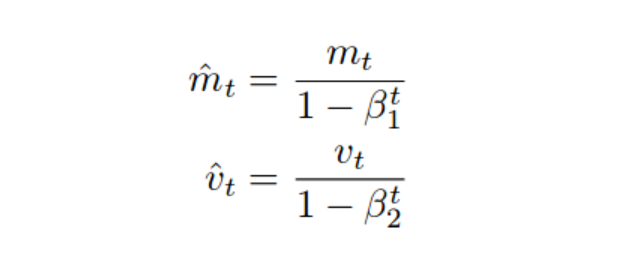In general we keep the values of β1 and β2 as 0.9 and 0.999.

Code:

The example code below demonstrates the use of different Optimizers while training different Artificial Neural Networks, the example code uses a Multilayer Perceptron(MLP) as a small neural network which is being trained using different Optimizers on the MNIST data. MNIST data is a large database of handwritten digits (0-9) which is used as a benchmark in many image processing systems. The Output Section displays the accuracy on both training and testing data using different Optimizers.

				
@file:DependsOn("org.jetbrains.kotlinx:kotlin-deeplearning-api:0.2.0")//Deeplearning api
//Important keyword to use s2
%use s2


				
// Some important import statements required for the code
import java.util.* // Accessing all classes and methods in Java
import kotlin.* // Accessing all classes and methods in Kotlin
import kotlin.jvm.JvmStatic // Specifies that an additional static method needs to be generated from this element if this is
// a function

/*Important modules required to be included from Kotlinx of Maven Repository
for creating and customizing your own neural network and then training it.*/

import org.jetbrains.kotlinx.dl.dataset.* // Accessing all the pre-available datasets
import org.jetbrains.kotlinx.dl.api.core.Sequential // Accessing the sequential layers for creating neural network models
import org.jetbrains.kotlinx.dl.api.core.layer.core.Dense // Accessing the dense layers
import org.jetbrains.kotlinx.dl.api.core.layer.core.Input // Accessing the input layers
import org.jetbrains.kotlinx.dl.api.core.layer.reshaping.Flatten // Accessing the flatten layers for converting a 2-D image to 1-D
// layer of units
import org.jetbrains.kotlinx.dl.api.core.loss.Losses // Accessing the loss functions available
import org.jetbrains.kotlinx.dl.api.core.metric.Metrics // Accessing the metrics available
// Importing the required Activation Functions needed in our model
import org.jetbrains.kotlinx.dl.api.core.activation.Activations.Relu // ReLU Activation
import org.jetbrains.kotlinx.dl.api.core.activation.Activations.Softmax // Softmax Activation
// Accessing different Optimizers
import org.jetbrains.kotlinx.dl.api.core.optimizer.RMSProp
import org.jetbrains.kotlinx.dl.api.core.optimizer.SGD
// Accessing the MNIST Dataset
import org.jetbrains.kotlinx.dl.dataset.mnist


				
// Creation of a very simple Multilayer Perceptron(MLP) model
val model = Sequential.of(
Input(28,28,1),  // Each input image is of size 28 * 28
Flatten(), // We flatten the image pixels into a flat layer of units to feed into upcoming layers.
Dense(300,Relu), // Dense Layer 1 with Relu Activation
Dense(100,Relu), // Dense Layer 2 with Relu Activation
Dense(10,Softmax) // Dense Layer 3 with Softmax Activation
//We have 10 units here as MNIST dataset has 10 classes (1...10)
)


				
val (train, test) = mnist() // loading the training and testing images
// Model is being compiled to be "fitted" onto the training dataset and subsequently evaluating on the testing dataset.
model.apply {
compile(
optimizer = Adam(), // Optimizer in use (User may choose any of the optimizers available depending on the problem)
loss = Losses.SOFT_MAX_CROSS_ENTROPY_WITH_LOGITS, // Cross-Entropy Loss
metric = Metrics.ACCURACY // Metric will be accuracy of the model
)
fit(dataset = train,epochs = 10) //You can think of the training process as "fitting" the model to describe the given data.
//epochs is basically the number of passes the model has to complete.
}


				
// Evaluating and printing the model performance (Accuracy)
val accuracy_test = model.evaluate(dataset = test).metrics[Metrics.ACCURACY]
val accuracy_train = model.evaluate(dataset = train).metrics[Metrics.ACCURACY]
println("Train Accuracy $accuracy_train") println("Test Accuracy$accuracy_test")



Output using different Optimizers:

SGD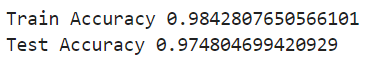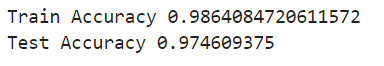RMS Prop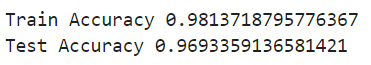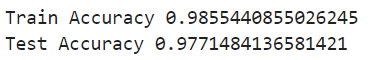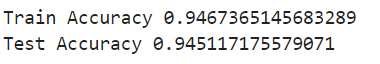Conclusion:

Batch gradient descent is guaranteed to converge to the global minimum for convex error surfaces and to a local minimum for non-convex surfaces if we correctly set our learning parameters.

But the main challenge comes when the search space created while training different Artificial Neural Networks is not in a good shape and contains Ravines and other irregularities which makes Gradient Descent difficult to converge to the global minimum. Different optimizers introduced in this article can help in better training of neural networks for different problems one may encounter. One may choose one optimizer over the other depending on the problem in hand as well as their experience.

As an example of Saddle Point in a search space, the below image represents the exploration paths of different optimizers in the search space: